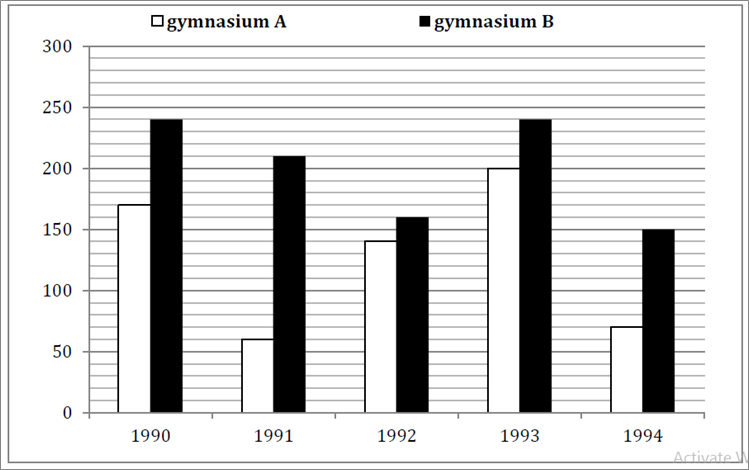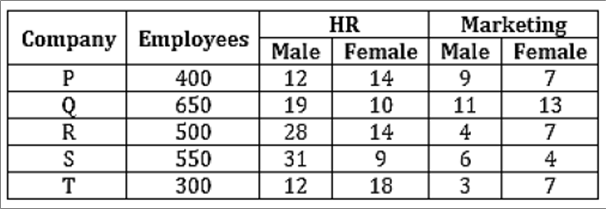sbi clerk :: numerical ability :: test 2

Home sbi clerk / numerical ability Questions and Answers

1.  Directions(Q.1 to Q.5 ):
In the Bar-chart, total members enrolled in different years from 1990 to 1994 in two gymnasium A and B. Based on this Bar chart solve the following questions-If in the year 1995 there is 30% increase in total number of members enrolled then in 1994 by both gymnasium, then find the total no. of members enrolled in 1995?

a

282

b

296

c

292

d

286

e

none of theseView AnswerDiscuss
 Answer : Option D Explanation : Required members = $\frac{{130}}{{100}} \times 220 = 286$

2.  The ratio between total members of both gymnasium in 1991 to total members in 1994 of both gymnasium is-

a

22:27

b

21:11

c

11:21

d

25:13

e

27:22View AnswerDiscuss
 Answer : Option E Explanation : Required Ratio = $\frac{{60 + 210}}{{70 + 150}} = \frac{{270}}{{220}} = 27:22$

3.  The number of members of gymnasium A in 1991 is what % of the no. of members of gymnasium B in 1994.

a

60%

b

55%

c

58%

d

62%

e

none of theseView AnswerDiscuss
 Answer : Option E Explanation : Required % = $\frac{{60}}{{150}} \times 100$ =20×2 =40%

4.  The total number of members enrolled in gymnasium A from 1991 to 1994 together is what percent more than the total number of members enrolled in gymnasium B in 1993 and 1994 together?(Rounded off to 2 decimal places)

a

10.51%

b

20.51%

c

15.51%

d

17.51%

e

none of theseView AnswerDiscuss
 Answer : Option B Explanation : Required % = $\frac{{\left( {60 + 140 + 200 + 70} \right) - \left( {240 + 150} \right)}}{{\left( {240 + 150} \right)}} \times 100$ $= \frac{{470 - 390}}{{390}} \times 100$ $= \frac{{80}}{{390}} \times 100$ $= 20.51{\rm{\% }}$

5.  Total member enrolled in gymnasium B in 1993 and 1994 together is what % more than members enrolled in gymnasium A in 1990 and 1994 together?

a

60%

b

65%

c

62.5%

d

61.5%

e

none of theseView AnswerDiscuss
 Answer : Option C Explanation : Required % = $\frac{{\left( {240 + 150} \right) - \left( {170 + 70} \right)}}{{\left( {170 + 70} \right)}} \times 100$ $= \frac{{150}}{{240}} \times 100 = 62.5{\rm{\% }}$

6.  Directions(Q.6 to Q.10 ):
What should come in place of question mark (?) in the following number series?

4,3,4,7,15, ?

a

38.5

b

40

c

45

d

37.5

e

none of theseView AnswerDiscuss
 Answer : Option A Explanation : ×0.5+1,×1+1,×1.5+1,×2+1……

7.  7,5,7,17,63, ?

a

321

b

309

c

305

d

301

e

none of theseView AnswerDiscuss
 Answer : Option B Explanation : ×1−2,×2−3,×3−4,×4−5,×5−6 ……

8.  11,14,19,28,43, ?

a

60

b

63

c

66

d

70

e

none of theseView AnswerDiscuss
 Answer : Option C Explanation : +3, +5, +9, +15……. +2, +4, +6……… 15+8=23, 43+23=66.

9.  2 60 10 120 30 ?

a

222

b

216

c

208

d

230

e

None of theseView AnswerDiscuss
 Answer : Option E Explanation : 1 3 +1, 4 3 −4, 2 3 +2, 5 3 −5, 3 3 +3, 6 3 −6 6 3 −6=210

10.  23 50 108 232 492 ?

a

1028

b

1024

c

1020

d

1032

e

None of theseView AnswerDiscuss
 Answer : Option A Explanation : Series is ×2+(4+4×0) ×2+(4+4×0+4×1) ×2+(4+4×0+4×1+4×2).. so on

11.  Directions(Q.11 to Q.15 ):
There are five companies and we have given the no. of employee working in different companies. In the table we have also given the percentage of male and female employees of HR and Marketing department.If 60% of the employees of company T in HR department have MBA degree and 40% of the employees of the same company in the Marketing dept. have MBA degree, then how many employees have MBA degree in company T in both dept. together.

a

98

b

108

c

106

d

92

e

66View AnswerDiscuss
 Answer : Option E Explanation : Required no. of employees $= \frac{{60}}{{100}} \times \frac{{30}}{{100}} \times 300 + \frac{{40}}{{100}} \times \frac{{10}}{{100}} \times 300$ $= 54 + 12 = 66$

12.  Find the ratio of female employees of company Q in HR dept. to male employees of company R in Marketing dept. ?

a

4:13

b

5:22

c

22:5

d

13:4

e

none of theseView AnswerDiscuss
 Answer : Option D Explanation : Required Ratio =65∶20 = 13 : 4

13.  Total number of HR employees of company P is what % more than the total no. of marketing employee in company T?

a

236.76%

b

226.67%

c

276.76%

d

246.67%

e

none of theseView AnswerDiscuss
 Answer : Option D Explanation : HR employees in company P = 26×4=104 Marketing employee in company T =10×3 = 30 Required % = $\frac{{104 - 30}}{{30}} \times 100 = 246.67{\rm{\% }}$

14.  The ratio of male employees in HR dept. of company P and R together to female employees of Marketing department in company S and T together?

a

187:27

b

43:188

c

188:43

d

27:187

e

none of theseView AnswerDiscuss
 Answer : Option C Explanation : Required Ratio = $\frac{{48 + 140}}{{22 + 21}} = \frac{{188}}{{43}} = 188:43$

15.  Difference between female employees of HR dept. in all companies together (excluding company S) and the female employees of Marketing dept. in all companies together (excluding company Q)?

a

139

b

129

c

135

d

141

e

none of theseView AnswerDiscuss
 Answer : Option A Explanation : Required difference =(56+65+70+54)−(28+35+22+21) =245−106=139Numerical AbilityEnglish LanguageReasoning
Copyright 2021 - Govt Exam Guru Made withfor Govt Jobs Aspirants. | Privacy Policy | Terms and Conditions | Contact us | Sitemap |XML